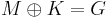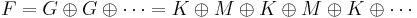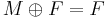# Solution May 21, 2012

M is projective, so there's some other module K such that$M \oplus K = G$ is free. Then,$F= G \oplus G \oplus \cdots = K \oplus M \oplus K \oplus M \oplus K \oplus \cdots$ is free, and$M \oplus F = F$.